Name:    2nd Grade Math Basic Test 5

Multiple Choice
Identify the choice that best completes the statement or answers the question.

1.The pencil is how many centimeters long?
 a. 5 cm d. 4 cm b. 6 cm e. 8 cm c. 7 cm

2.

Measurement – Money – RIT 171 – 180The value of the money is:
 a. \$2.00 d. \$4.00 b. \$1.00 e. \$30.00 c. \$3.00

3.

Which set of coins has the value of 63 cents?
 a.b.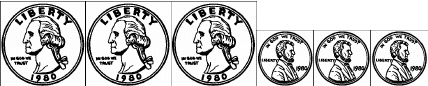c.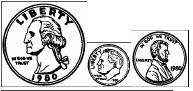d.e.4.

Measurement – Time, Temperature – RIT 171 – 180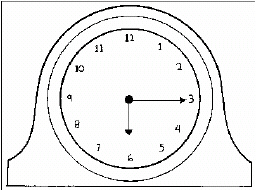a. 6:30 d. 5:15 b. 6:45 e. 3:06 c. 6:15

5.

What time is it?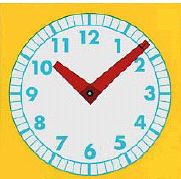a. 10:08 d. 1:10 b. 10:02 e. 11:08 c. 1:52

6.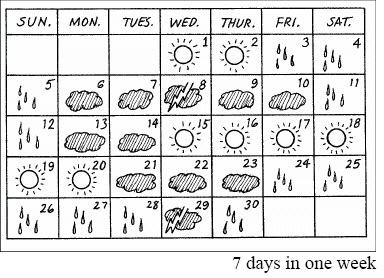How many days in 3 weeks?
 a. 10 d. 4 b. 21 e. 3 c. 7

7.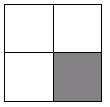What part of this square is shaded?
 a. 1/5 d. 1/3 b. 1/2 e. 1/6 c. 1/4

8.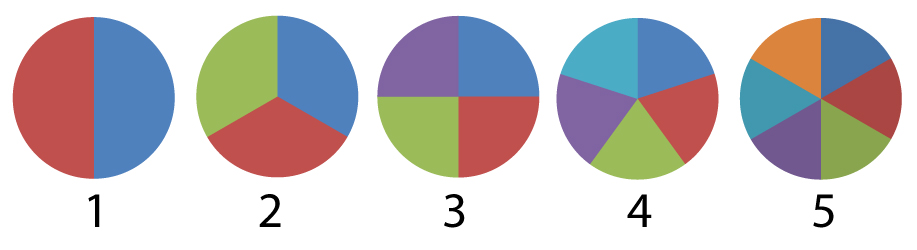Which pie has 3 equal parts?
 a. 1 d. 4 b. 2 e. 5 c. 3

9.

Number Sense and Numeration – Ordering, Equalities, and Inequalities –RIT 151 – 160a. 6, 8, 7 d. 7, 8, 6 b. 7, 6, 8 e. 6, 7, 8 c. 8, 6, 7

10.

Number Sense and Numeration – Whole Numbers – RIT 151 – 160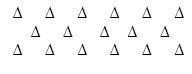How many?
 a. 12 d. 18 b. 16 e. 20 c. 17

11.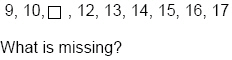a. 13 d. 17 b. 18 e. 12 c. 11

12.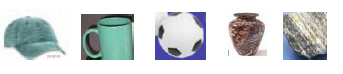Which item is third?
 a.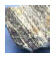d.b.e.c.13.

Number Sense and Numeration – Fractions – RIT 161 – 170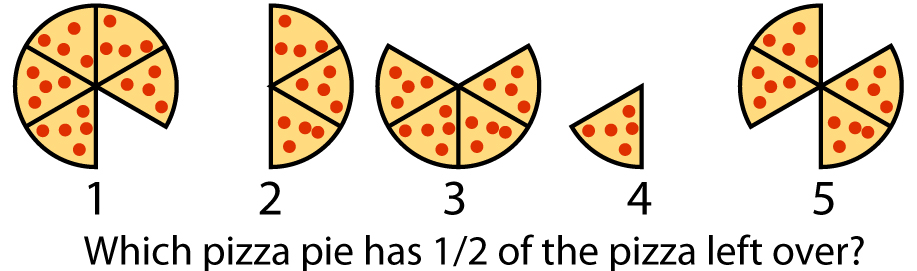a. 1 d. 4 b. 2 e. 5 c. 3

14.

Number Sense and Numeration – Ordering, Equalities, and Inequalities –RIT 161 – 170
Put the numbers in order from least to greatest.
78, 46, 87, 54
 a. 54, 78, 46, 87 d. 46, 54, 78, 87 b. 54, 78, 46, 87 e. 87, 78, 46, 54 c. 46, 54, 87, 78

15.

Number Sense and Numeration – Place Value – RIT 161 – 170
247
What is the value of the 4 in this number?
 a. 4 d. 40 b. 400 e. 4,000 c. 40,000